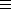## CGAL::Box_intersection_d::Box_with_handle_d<NT, int D, Handle, IdPolicy>

### Definition

Box_intersection_d::Box_with_handle_d<NT, int D, Handle, IdPolicy> is a generic iso-oriented bounding box in dimension D that stores additionally a handle to some underlying geometric object. It provides in each dimension an interval with lower and upper endpoints represented with the number type NT. This class is designed to work smoothly with the algorithms for intersecting sequences of iso-oriented boxes. For degeneracy handling, the boxes need to provide a unique id-number. The policy parameter IdPolicy offers several choices. The template parameters have to comply with the following requirements:

• NT: number type for the box boundaries, needs to be a model of the Assignable and the LessThanComparable concept.
• int D: the dimension of the box.
• Handle concept, e.g., a pointer, an iterator, or a circulator.
• IdPolicy: specifies how the id-number will be provided. Can be one of the following types, where ID_EXPLICIT is the default for this parameter:
• ID_NONE: no id-number is provided. Can be useful to have this class as a base class for different implementations of id-numbers than the ones provided here.
• ID_EXPLICIT: the id-number is stored explicitly in the box and automatically created and assigned at construction time of the box. Note that copying a box (copy-constructor and assignment) does not create a new id-number but keeps the old one, which is the behavior needed by the CGAL::box_self_intersection algorithm. This is therefore the safe default implementation.
• ID_FROM_BOX_ADDRESS: casts the address of the box into a std::ptrdiff_t to create the id-number. Works fine if the intersection algorithms work effectively with pointers to boxes, but not in the case where the algorithms work with box values, because the algorithms modify the order of the boxes, and the CGAL::box_self_intersection algorithm creates copies of the boxes that would not have identical id-numbers.
• ID_FROM_HANDLE: casts the address of the value of the handle into a std::ptrdiff_t to create the id-number. Works in many conceivable settings, e.g., it works with boxes copied by value or by pointer, and the self intersection test. It will not work if there is no one-to-one mapping between boxes and the geometry that is referred to with the handles, i.e., this id-number scheme fails if a geometric object creates several boxes with the same handle value. Note that this option is not available for the CGAL::Box_intersection_d::Box_d type that does not store a handle.

#include <CGAL/Box_intersection_d/Box_with_handle_d.h>

and also automatically with
#include <CGAL/box_intersection_d.h>

### Types

 Box_intersection_d::Box_with_handle_d::NT number type to represent the box boundaries. Allowed are the built-in types int, unsigned int, float, and double.

 typedef std::size_t ID; type for the box id-number.

### Creation

Box_intersection_d::Box_with_handle_d<NT, int D, Handle, IdPolicy> box;
Default constructor. No particular initialization.

Box_intersection_d::Box_with_handle_d<NT, int D, Handle, IdPolicy> box ( bool complete, Handle h);
initializes to the complete or the empty space. If empty, all interval starting(end) points will be set to positive(negative) infinity, sets handle to h.

Box_intersection_d::Box_with_handle_d<NT, int D, Handle, IdPolicy> box ( NT lo[D], NT hi[D], Handle h);
initializes the box intervals to [lo[i],hi[i]], 0i < D and sets the handle to h.
 Precondition: lo[i] < hi[i] for 0i < D.

Box_intersection_d::Box_with_handle_d<NT, int D, Handle, IdPolicy> box ( Bbox_2 bbox, Handle h);
constructs from bbox and sets the handle to h, exists iff D=2 and NTdouble.

Box_intersection_d::Box_with_handle_d<NT, int D, Handle, IdPolicy> box ( Bbox_3 bbox, Handle h);
constructs from bbox and sets the handle to h, exists iff D=3 and NTdouble.

### Modifiers

 void box.init ( bool complete = false) initializes to the complete or the empty space. If empty, all interval starting(end) points will be set to positive(negative) infinity. void box.extend ( NT point[D]) extend box to contain the old box and point.

### Access Functions

 Handle box.handle () returns the handle stored in box. int Box_with_handle_d::dimension () returns D, the dimension of the box. std::size_t box.id () returns a unique box id, see the IdPolicy template parameter above for the different choices. Does not exist if ID_NONE has been chosen for the IdPolicy. NT box.min_coord ( int d) returns the lower boundary in dimension d, 0d < D. NT box.max_coord ( int d) returns the upper boundary in dimension d, 0d < D. Bbox_2 box.bbox () returns the bounding box iff D=2 and NTdouble. Bbox_3 box.bbox () returns the bounding box iff D=3 and NTdouble.